# RD Sharma Class 10 Solutions Chapter 5 Arithmetic Progressions Ex 5.2

## RD Sharma Class 10 Solutions Chapter 5 Arithmetic Progressions Ex 5.2

These Solutions are part of RD Sharma Class 10 Solutions. Here we have given RD Sharma Class 10 Solutions Chapter 5 Arithmetic Progressions Ex 5.2

Other Exercises

Question 1.
Show that the sequence defined by an = 5n – 7 is an A.P., find its common difference.
Solution: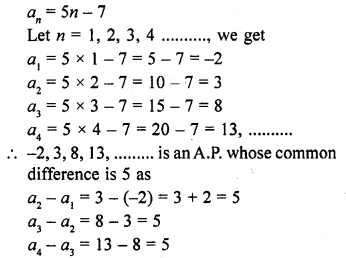Question 2.
Show that the sequence defined by an = 3n² – 5 is not an A.P.
Solution: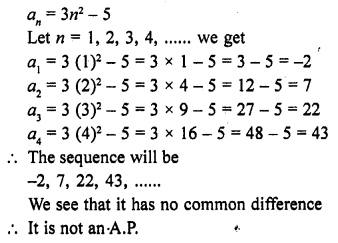Question 3.
The general term of a sequence is given by an = -4n + 15. Is the sequence an A.P.? If so, find its 15th term and the common difference.
Solution:
General term of a sequence
an = -4n + 15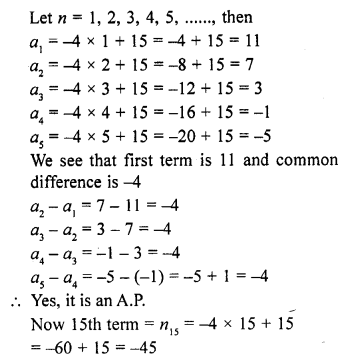Question 4.
Write the sequence with nth term :
(i) an = 3 + 4n
(ii) an = 5 + 2n
(iii) an = 6 – n
(iv) an = 9 – 5n
Show that all of the above sequences form A.P.
Solution: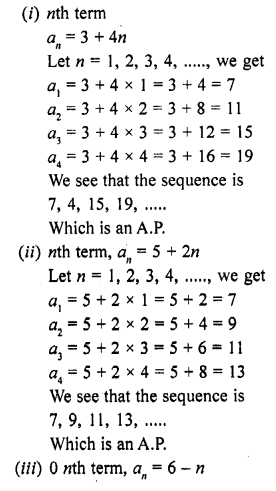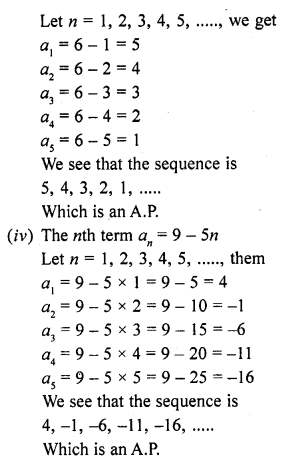Question 5.
The nth term of an A.P. is 6n + 2. Find the common difference. [CBSE 2008]
Solution: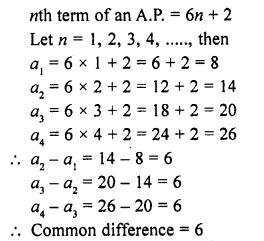Question 6.
Justify whether it is true to say that the sequence, having following nth term is an A.P.
(i) an = 2n – 1
(ii) an = 3n² + 5
(iii) an = 1 + n + n²
Solution: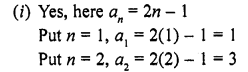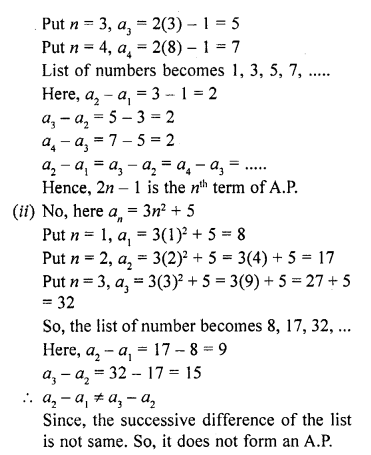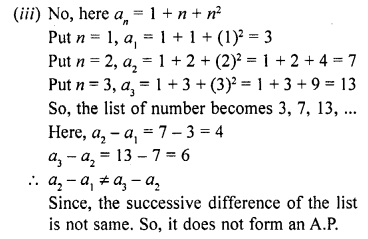Hope given RD Sharma Class 10 Solutions Chapter 5 Arithmetic Progressions Ex 5.2 are helpful to complete your math homework.

If you have any doubts, please comment below. Learn Insta try to provide online math tutoring for you.